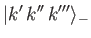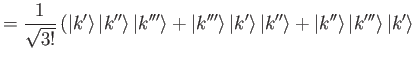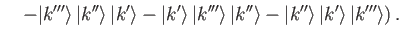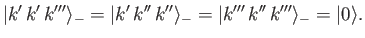Next: Two-Electron System Up: Identical Particles Previous: Permutation Symmetry

# Spin Statistics Theorem

We have seen that the exchange degeneracy of a system of identical particles is such that a specification of a complete set of observable eigenvalues does not uniquely determine the corresponding state ket. However, we have also demonstrated that there are only two possible state kets: that is, a ket that is totally symmetric with respect to particle interchange, or a ket that is totally antisymmetric. It turns out that systems of identical particles possessing integer-spin (e.g., spin 0, or spin 1) always choose the totally symmetric ket, whereas systems of identical particles possessing half-integer-spin (e.g., spin 1/2) always choose the totally antisymmetric ket. This additional piece of information--which is generally known as the spin statistics theorem--ensures that the specification of a complete set of observable eigenvalues of a system of identical particles does, in fact, uniquely determine the corresponding state ket.

Systems of identical particles whose state kets are totally symmetric with respect to particle interchange are said to obey Bose-Einstein statistics [14,39]. Moreover, such particles are termed bosons. On the other hand, systems of identical particles whose state kets are totally antisymmetric with respect to particle interchange are said to obey Fermi-Dirac statistics [43,28], and the constituent particles are called fermions. In non-relativistic quantum mechanics, the rule that all integer-spin particles are bosons, whereas all half-integer spin particles are fermions, must be accepted as an empirical fact. However, in relativistic quantum mechanics, it is possible to formulate reasonably convincing arguments that half-integer-spin particles cannot be bosons, and integer-spin particles cannot be fermions [47,81]. Incidentally, electrons, protons, and neutrons are all fermions.

The Pauli exclusion principle  is an immediate consequence of the fact that electrons obey Fermi-Dirac statistics. This principle states that no two electrons in a multi-electron system can possess identical sets of observable eigenvalues. For instance, in the case of a three-electron system, the state ket is(9.42)

[See Equation (9.41).] Note, however, that(9.43)

In other words, if two of the electrons in the system possess the same set of observable eigenvalues then the state ket becomes the null ket, which corresponds to the absence of a state.Next: Two-Electron System Up: Identical Particles Previous: Permutation Symmetry
Richard Fitzpatrick 2016-01-22